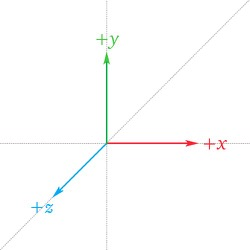Euler angles are three angles used to describe the orientation of a rigid body, they are typically denoted $$\alpha, \beta, \gamma$$, these angles represent a sequence of three elemental rotations about the axes of some coordinate system

## Intrinsic and extrinsic rotations

### Intrinsic rotations

A set of intrinsic rotations represent rotations relative to the object space which changes after each rotation

If the axes of some coordinate system are $$X,Y,Z$$ (note that initially the axes are aligned with the axes of a fixed coordinate system $$x,y,z$$), one of the most conventional set of intrinsic rotations is $$z-x'-z''$$, it's computed as follows

• perform a rotation of $$\alpha$$ around the $$z$$-axis, the resulting set of axes is $$x', y', z'$$ (note that $$z' = z$$)
• perform a rotation of $$\beta$$ around the $$x'$$-axis, the resulting set of axes is $$x'', y'', z''$$ (note that $$x'' = x'$$)
• perform a rotation of $$\gamma$$ around the $$z''$$-axis, the resulting set of axes is $$x''', y''', z'''$$ (note that $$z''' = z''$$ and that the object space $$z$$-axis is used twice in the overall rotation)intrinsic rotation$$z-x'-z''$$, note that the$$+z$$-axis points upward, the$$+x$$-axis points left and the$$+y$$-axis point right (all shown in blue), the rotated system$$X,Y,Z$$is shown in red

A rotation matrix (used to pre-multiply column vectors) can be used to represent a sequence of intrinsic rotations, for example the extrinsic rotations $$x-y'-z''$$ with angles $$\alpha, \beta, \gamma$$ are represented as a multiplication of the following rotation matrices

$\mathbf{R} = \mathbf{X}(\alpha)\mathbf{Y}(\beta)\mathbf{Z}(\gamma)$

Where $$\mathbf{X}(\alpha)$$, $$\mathbf{Y}(\beta)$$ and $$\mathbf{Z}(\gamma)$$ are rotation matrices that represent a rotation around the $$x$$-axis by $$\alpha$$, around the $$y$$-axis by $$\beta$$ and around the $$z$$-axis by $$\gamma$$ respectively

### Extrinsic rotations

A set of extrinsic rotations represent rotations relative to a fixed coordinate system (typically the world coordinate system), for example the set of extrinsic rotations $$z-x-z$$ works as follows

• perform a rotation of $$\alpha$$ around the $$z$$-axis, the resulting set of axes is $$x', y', z'$$ (note that $$z' = z$$)
• perform a rotation of $$\beta$$ around the $$x$$-axis, the resulting set of axes is $$x'', y'', z''$$
• perform a rotation of $$\gamma$$ around the $$z$$-axis, the resulting set of axes is $$x''', y''', z'''$$

A rotation matrix (used to pre-multiply column vectors) can be used to represent a sequence of intrinsic rotations, for example the extrinsic rotations $$x-y-z$$ with angles $$\alpha, \beta, \gamma$$ are represented as a multiplication of the following rotation matrices

$\mathbf{R} = \mathbf{Z}(\gamma)\mathbf{Y}(\beta)\mathbf{X}(\alpha)$

Where $$\mathbf{X}(\alpha)$$, $$\mathbf{Y}(\beta)$$ and $$\mathbf{Z}(\gamma)$$ are rotation matrices that represent a rotation around the $$x$$-axis by $$\alpha$$, around the $$y$$-axis by $$\beta$$ and around the $$z$$-axis by $$\gamma$$ respectively

### Conversion between intrinsic rotations and extrinsic rotations

Any intrinsic rotation is equivalent to an extrinsic rotation by the same angles but with inverted order of rotations

For example the intrinsic rotations $$x-y'-z''$$ by the angles $$\alpha,\beta,\gamma$$ are equivalent to the extrinsic rotations $$z-y-x$$ by the angles $$\gamma,\beta,\alpha$$, both represented by

$\mathbf{R} = \mathbf{X}(\alpha)\mathbf{Y}(\beta)\mathbf{Z}(\gamma)$

## Proper Euler angles

A sequence of three elemental rotations are called proper Euler angles when the first and third rotation axes are the sameProper Euler angles representing rotations about$$z-x'-z''$$by the angles$$\alpha, \beta, \gamma$$, the rotated system$$X,Y,Z$$is shown in red

There are six possibilities of choosing the rotation axes for proper Euler angles which are intrinsic rotations in a similar way there are other six other possibilities of choosing the rotation axes which are extrinsic rotations

intrinsic rotations extrinsic rotations
$$x-y'-x''$$ $$x-y-x$$
$$x-z'-x''$$ $$x-z-x$$
$$y-x'-y''$$ $$y-x-y$$
$$y-z'-y''$$ $$y-z-y$$
$$z-x'-z''$$ $$z-x-z$$
$$z-y'-z''$$ $$z-y-z$$

## Tait-Bryan angles

A sequence of three elemental rotations are called Tail-Bryan angles when the angles represent rotations about three distinct axes

Just like proper Euler angles there are 6 possible intrinsic rotations and 6 possible extrinsic rotations

intrinsic rotations extrinsic rotations
$$x-y'-z''$$ $$z-y-x$$
$$x-z'-y''$$ $$y-z-x$$
$$y-x'-z''$$ $$z-x-y$$
$$y-z'-x''$$ $$x-z-y$$
$$z-x'-y''$$ $$y-x-z$$
$$z-y'-x''$$ $$x-y-z$$

The set of intrinsic rotations $$z-y'-x''$$ is known as yaw, pitch and roll, these angles are also known as nautical angles because they can describe the orientation of a ship or aircraftTait–Bryan angles representing the sequence$$z-y'-x''$$

The rotation matrix for the sequence $$z-y'-x''$$ (or $$x-y-z$$) which is known as yaw, pitch and roll is given by

\begin{align*} \mathbf{R} = \mathbf{Z}(\alpha)\mathbf{Y}(\beta)\mathbf{X}(\gamma) \end{align*}

## Extrinsic rotations expressed in upright space

An important thing to note is that the standard rotation matrices work in upright space, if the object space axes are not aligned with the upright space axes (different direction) then the sequence of extrinsic rotations must be done on the axes expressed in upright space

For example given that world space isChosen world space$$+x$$(right),$$+y$$(up) and$$+z$$(backward), note that the choice is just personal preference

If there's an object whose object space axes $$+x$$ (backward), $$+y$$ (right) and $$+z$$ (up) then a sequence of intrinsic rotations $$z-y'-x''$$ by the angles $$\alpha, \beta, \gamma$$ (equivalent to the extrinsic rotation $$x-y-z$$ by the angles $$\gamma, \beta, \alpha$$ which is also known as yaw, pitch and roll) is equivalent to the multiplication of the following rotation matrices

$\mathbf{R} = \mathbf{R}(\mathbf{w}, \alpha)\mathbf{R}(\mathbf{v}, \beta)\mathbf{R}(\mathbf{u}, \gamma)$

Where

• $$\mathbf{R}(\mathbf{s}, t)$$ is the general rotation matrix used to rotate around the axis $$\mathbf{s}$$ by the angle $$t$$
• $$\mathbf{u, v, w}$$ are the columns of the transformation matrix used to transform any point $$\mathbf{p}$$ expressed in object space to upright space

\begin{align*} \mathbf{p}_{upright} &= \mathbf{M}_{upright \leftarrow object} \mathbf{p}_{object} \\ &= \begin{bmatrix} \mathbf{u}_{3 \times 1} \mathbf{v}_{3 \times 1} \mathbf{w}_{3 \times 1}\end{bmatrix} \mathbf{p}_{object} \end{align*}

The problem can be simplified when frame is somewhat aligned with the upright space (the order might be different and the axis directions might be reversed but it's still aligned), the following diagram shows some of these simplifications